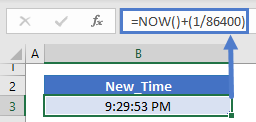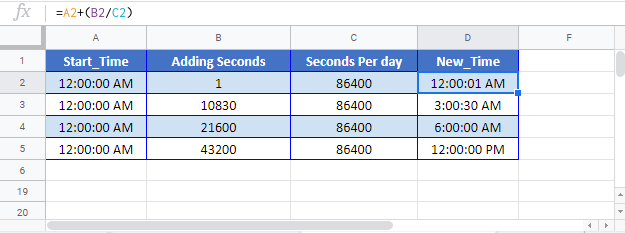This tutorial will demonstrate how to add seconds to a time in Excel and Google Sheets.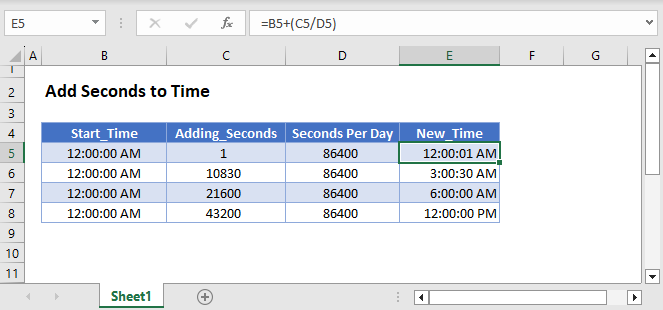In Excel, time is stored as a fractional value where each 1/24th represents one hour of the day. So one second is 1/24/60/60 = 1/86400.

``=B3/86400``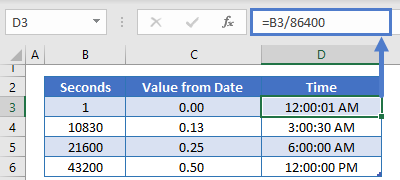``=B3+(1/86400)``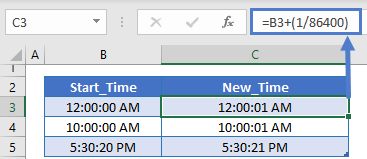or 59/86400 to add 59 seconds:

``=B3+(59/86400)``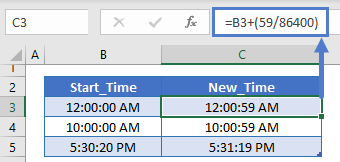To add a second to the current time, add 1/86400 to the NOW Function:

``=NOW()+(1/86400)``